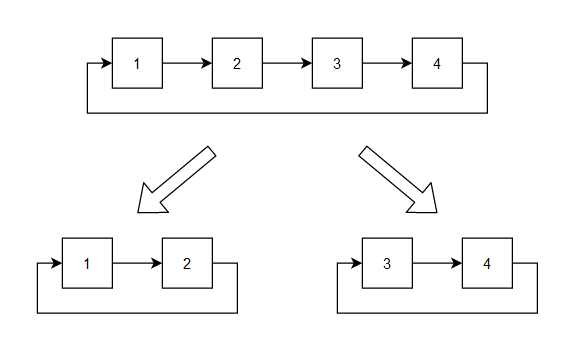# Split A Circular Linked List

Posted: 6 Jan, 2021
Difficulty: Easy

## PROBLEM STATEMENT

#### For Example :

``````Let the circular linked list be 1, 2, 3, 4. We have to split this into two equal-sized circular linked lists.
``````##### Input format :
``````The first line of input contains an integer ‘T’, denoting the number of test cases.

The first line of each test case contains an integer ‘N’, denoting the number nodes in the circular linked list.

The second line of each test case contains ‘N’ space-separated integers denoting the elements of the circular linked list.
``````
##### Output format :
``````For each test case, both the circular linked lists are printed in separate lines after splitting.

The output for each test case is printed in a separate line.
``````
##### Note :
``````You do not need to print anything, it has already been taken care of. Just implement the given function.
``````
##### Constraints :
``````1 <= T <= 10
2 <= N <= 10000, N is even
-10 ^ 9 <= node data <= 10 ^ 9

Where  ‘node data’ is the value of nodes of the list.

Time limit: 1 sec
``````Approach 1

The simple approach would be finding the middle point of the circular linked list and changing the pointers.

• Find the middle point of the circular linked list by first counting the number of nodes in the circular linked list then traversing half of them from the head.
• To make the second half of the circular linked list connect the last node, which is just before head, to the next of the middle node of the circular linked list.
• To make the first half of the circular linked list connect the middle node of the circular linked list to the head of the circular linked list.